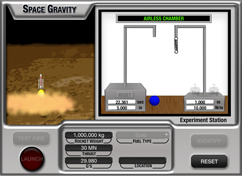# Space GravityMost students know that gravity near the surface of Earth accelerates objects downward at 32 feet/sec2. That means, in the absence of air after an object starting at rest has fallen for 1 second, its speed would be 32 feet/sec. After two seconds of falling, the object would have a speed of 64 feet/sec. Can you determine the value of gravitational acceleration for objects on other planetary objects? Don’t drop the ball on this one. Hummm, maybe you should.

### What is gravitational acceleration?

The gravitational acceleration of an object is the rate at which the object accelerates due to the mass of another object. On Earth's surface in the absence of air, all objects accelerate at about 9.8 m/s2 (meters per second squared) due to the large mass of Earth. An object dropped on the moon's surface would accelerate at a lower rate due to the moon's smaller mass and smaller gravitational force.

### What is speed?

Speed is defined as the distance an object travels in an elapsed time. A car that travels 50 miles in 2 hours would have a speed of 25 miles per hour.

### What is Hooke's law?

Hooke's law describes how springs and other similar elastic materials behave when stretched or compressed. Hooke's law states that the more a spring is stretched, the greater the restoring force of the spring is. The law also relates how a characteristic of the spring, called stiffness, affects the restoring force. Hooke's law can be described with the following equation: F = -kx (where F = restoring force, k = spring constant, and x is the stretch distance) The equation has a negative sign (-) because the restoring force is always in the direction opposite to the stretch. Also, Hooke's law is only valid until the elastic limit of the spring is reached. Once the spring has been stretched beyond the elastic limit, Hooke's law can no longer be used to describe the restoring force.

### What is a spring constant?

The spring constant, symbolized by the letter "k," describes the stiffness of the spring. A large spring constant value indicates a stiffer spring than a spring with a lower spring constant. The spring constant is a measure of the force required to stretch or compress a spring a given distance.

### What is rocket thrust?

The thrust of a rocket engine is a measure of the force exerted on a rocket by the engine as a result of Newton's third law of motion. The greater the engine thrust, the larger the force applied to push the rocket forward. Thrust is achieved by the combustion reaction of various rocket fuels.

### What are "g's?"

The term "g's" refers to an acceleration felt as weight. At Earth's surface, humans are said to experience an acceleration of 1 g, although their actual acceleration is zero since they are not moving vertically. A skydiver does have a 1 g acceleration; however, the person feels weightless because she does not feel a supporting force holding her body against the earth. A person at the bottom of a loop on a rollercoaster may feel 4 g's, which pushes the person down hard into the seat and makes him feel four times heavier than normal.

### How many "g's" can the human body withstand?

The number of g's a human can tolerate is highly dependent on the length of time the acceleration is experienced, the direction of the acceleration, and on personal characteristics of the human undergoing the acceleration. Although people can withstand accelerations of over 50 g's for fractions of a second, more than 25 g's for an extended number of seconds are usually fatal. Many people pass out when exposed to between 3 to 4 g's for more than a couple of seconds.